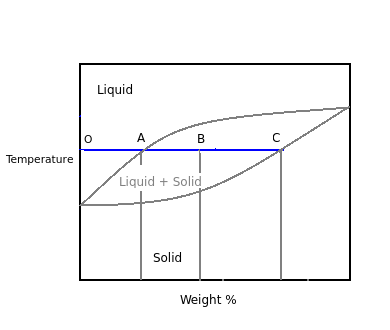# Why is Lever rule used?

Q.  The rule used to determine composition of various phases in a phase diagram is _____
- Published on 11 Sep 15

a. Gibb's phase rule
b. Lever rule
c. Both a. and b.
d. None of the above

#### Discussion

• Sravanthi   -Posted on 20 Nov 15
- The rule used to determine composition of various phases in a phase diagram is Lever rule.

- This rule is used to determine weight percentage of each phase of a binary equilibrium phase diagram.- The relation between arms of lever and amount of phases according to Lever's rule is given as:

- (Amount of solid) / (Amount of liquid) = Arm AB / Arm BC

- Therefore, by considering weight at points A, B and B, C. The amount of liquid in percentage can be calculated by substituting the values of weight.

## ➨ Post your comment / Share knowledgeEnter the code shown above:

(Note: If you cannot read the numbers in the above image, reload the page to generate a new one.)